Algebra 1 7-5 Complete Lesson: Rational Exponents and Radicals
starstarstarstarstarstarstarstarstarstar
4.5 (1 rating)
by Matthew Richardson
| 45 Questions
Note from the author:
A complete formative lesson with embedded slideshow, mini lecture screencasts, checks for understanding, practice items, mixed review, and reflection. I create these assignments to supplement each lesson of Pearson's Common Core Edition Algebra 1, Algebra 2, and Geometry courses. See also mathquest.net and twitter.com/mathquestEDU.The outlined content above was added from outside of Formative.1
1
10 pts
Solve It! The figure is made up of squares. The side of a larger square is bisected by the vertices of the square that is one size smaller. What are the values of a, b, and c? Hint: The hypotenuse of an isosceles right triangle equals the smaller side times the square root of 2.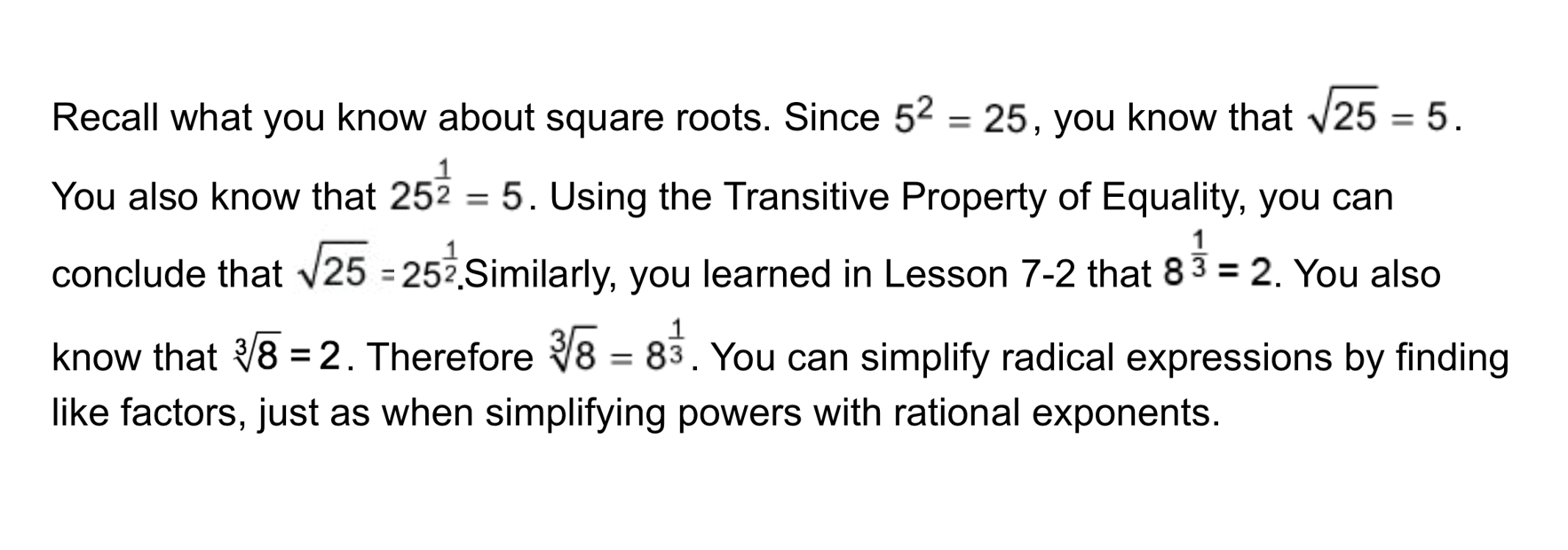2
2
10 pts
Problem 1 Got It?
A
B
C
D3
3
10 pts
Problem 1 Got It?
A
B
C
D4
4
10 pts
Problem 1 Got It?
A
B
C
D5
5
10 pts
Problem 1 Got It?
A
B
C
D6
6
10 pts
Problem 2 Got It?
A
B
C
D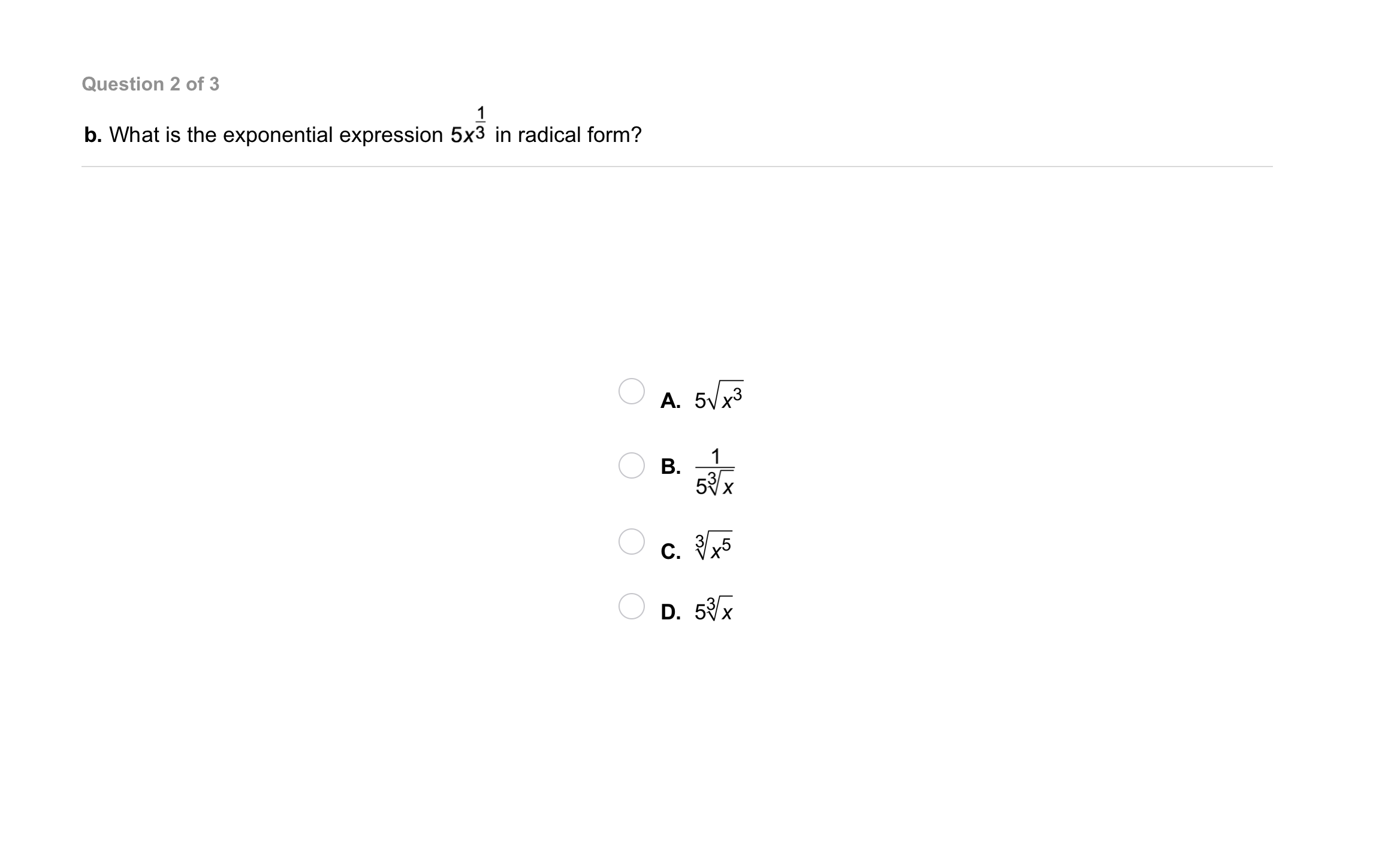7
7
10 pts
Problem 2 Got It?
A
B
C
D8
8
10 pts
Problem 2 Got It?
A
B
C
D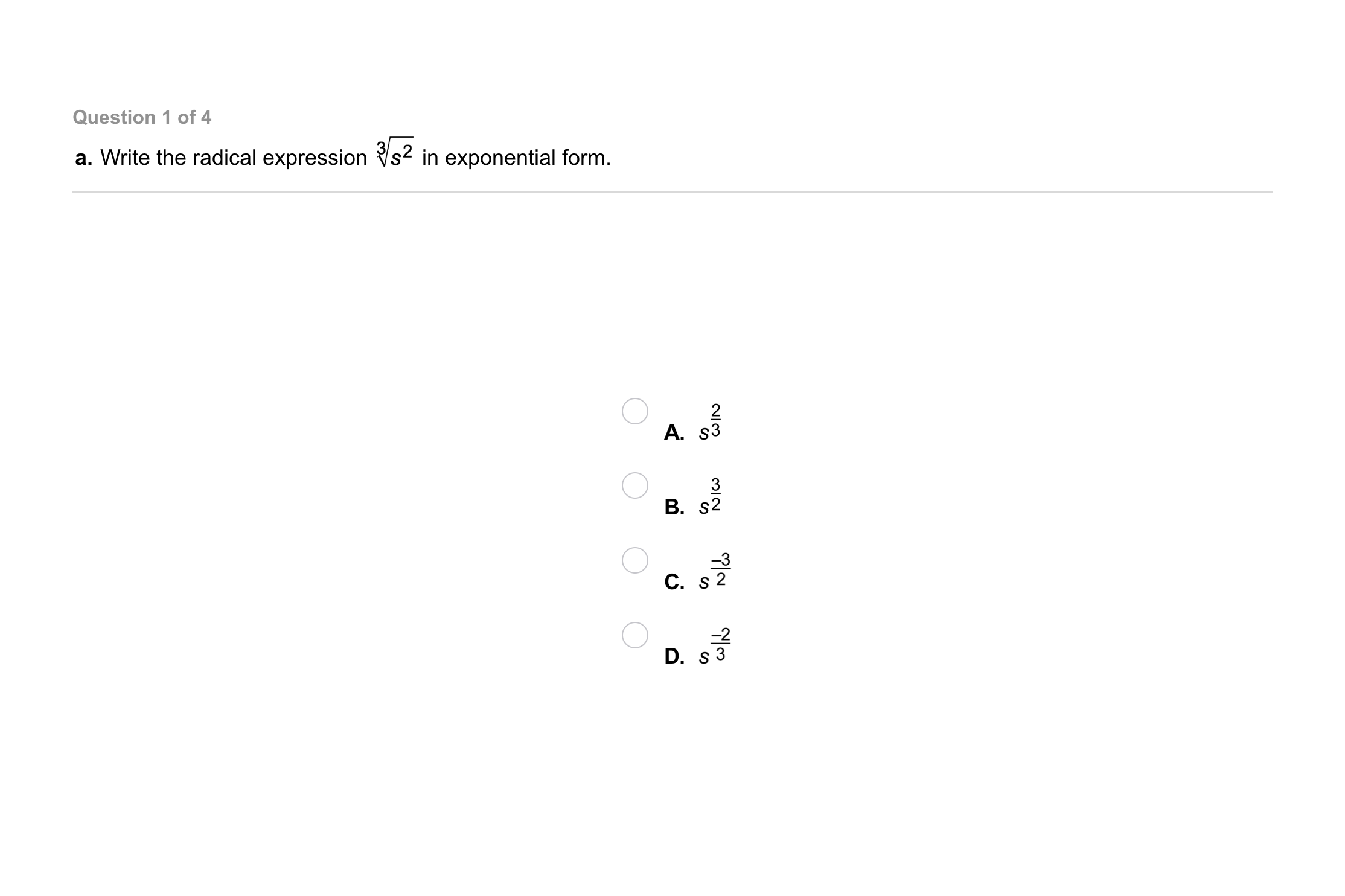9
9
10 pts
Problem 3 Got It?
A
B
C
D10
10
10 pts
Problem 3 Got It?
A
B
C
D11
11
10 pts
Problem 3 Got It?
A
B
C
D12
12
10 pts
Problem 3 Got It?
A
B
C
D13
13
10 pts
Problem 4 Got It?
A
B
C
D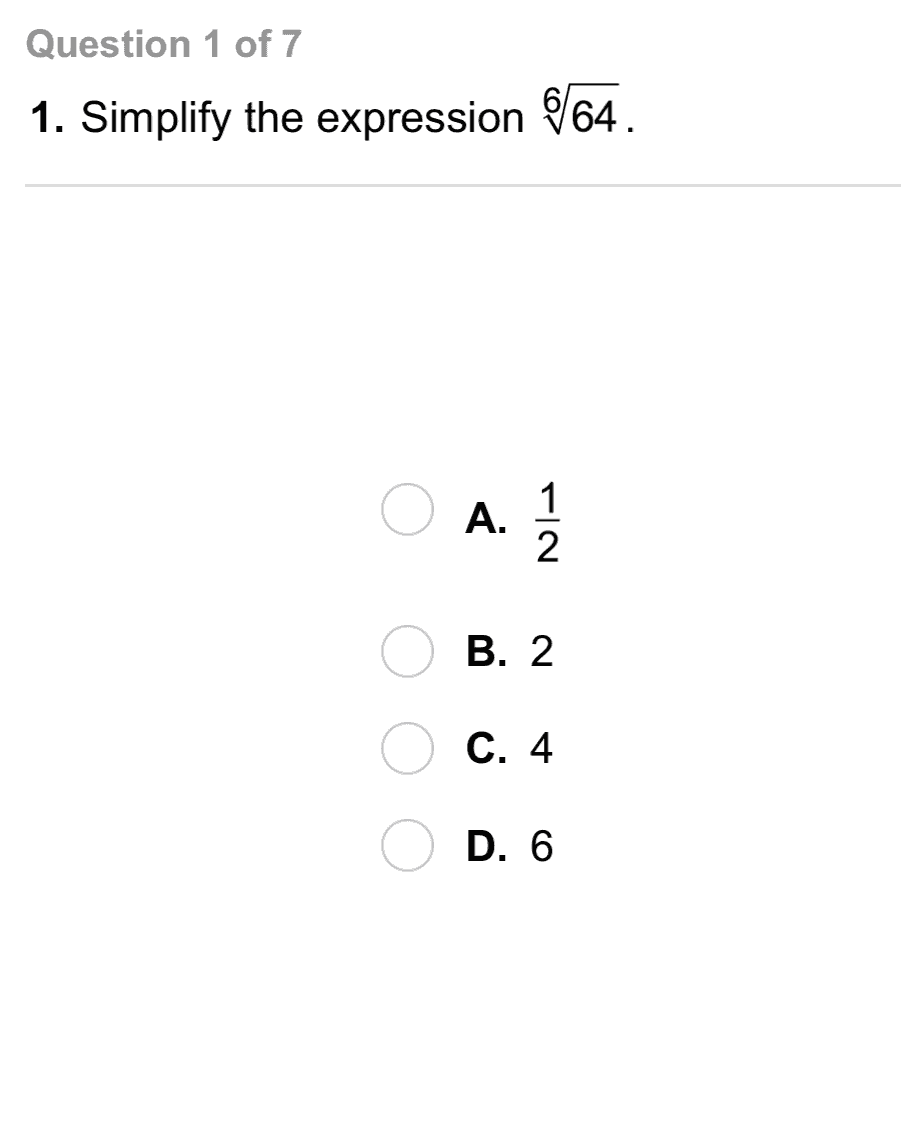14
14
10 pts
A
B
C
D15
15
10 pts
A
B
C
D16
16
10 pts
A
B
C
D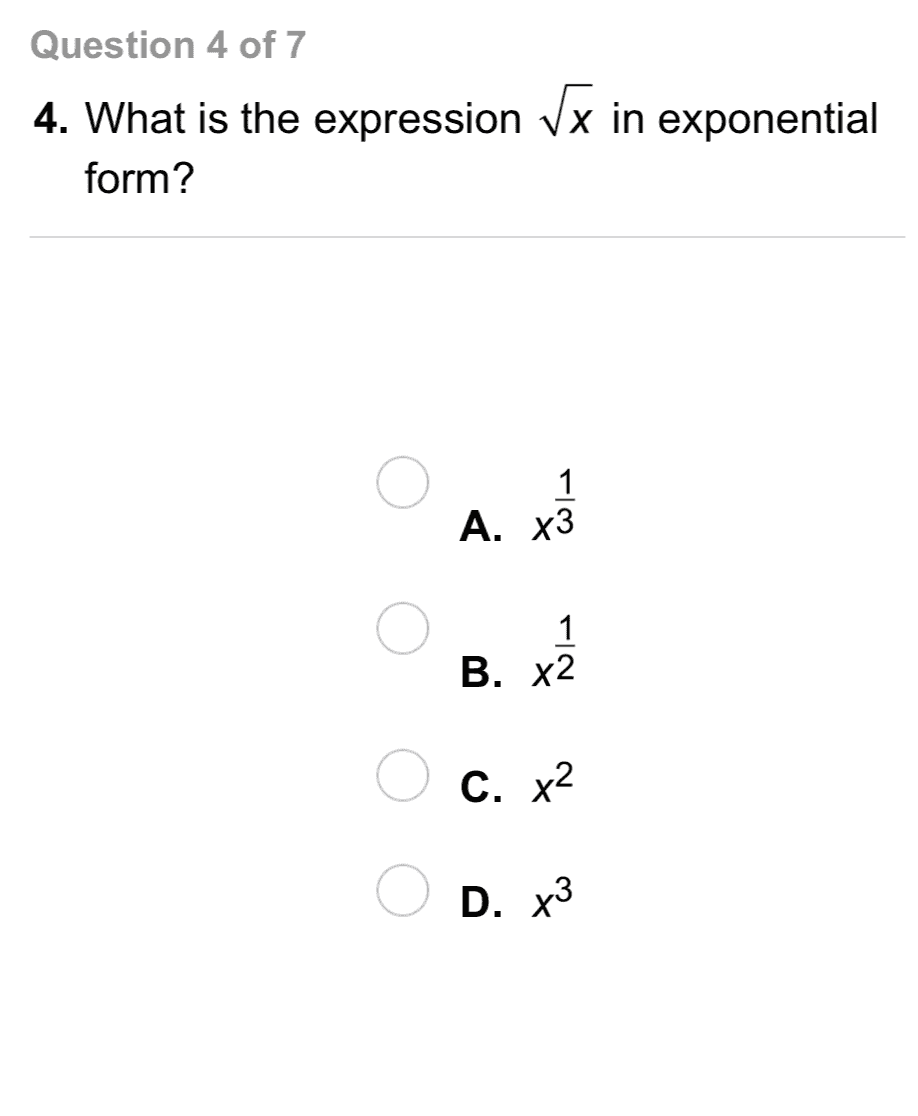17
17
10 pts
A
B
C
D18
18
10 pts
A
B
C
D19
19
10 pts
A
B
C
D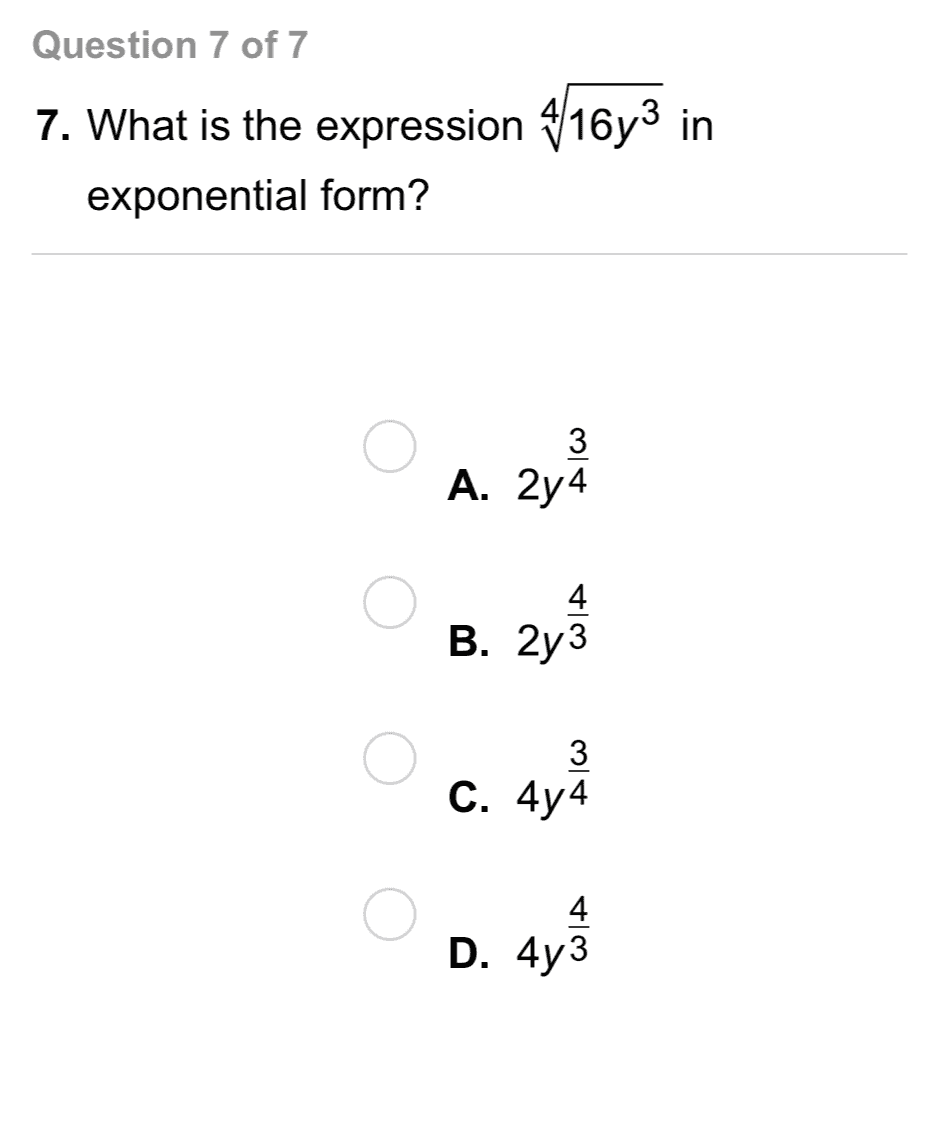20
20
10 pts
A
B
C
D21
22
21
10 pts
Error Analysis: What is the error in the problem shown?
22
10 pts
What is the correct simplified form?
23
20 pts
Reasoning: Write a rule for multiplying two radicals with the same radicand. Justify why your rule works.
24
5 pts
Reasoning: Is this equation true?

No, it is false.
Yes, it is true.26
5 pts
Review Lesson 7-3: Simplify the expression.
27
5 pts
Review Lesson 7-3: Simplify the expression.
28
5 pts
Review Lesson 7-3: Simplify the expression.
29
5 pts
Review Lesson 7-3: Simplify the expression.
30
5 pts
Review Lesson 7-3: Simplify the expression.
31
10 pts
Review Lesson 5-5: Identify the form of each equation.
• slope-intercept form
• point-slope form
• standard form
• none of these
• 5x + 3y = 21
• y = -7x + 1
• x = 5 + 3y
• y = -9(x + 2)
32
10 pts
Review Lesson 5-5: Is the equation in standard form? Explain.
Yes, the equation is in standard form. It is in the form Ax + By = C, A, B, and C are real numbers, and A and B are not both zero.
No, the equation is not in standard form. Standard form is y = mx + b.
No, the equation is not in standard form. -2 is not a real number.
33
10 pts
Review Lesson 5-5: Match each equation from the left column with its equivalent standard form equation in the right column.
• y = 4x + 7
• y + 3 = 2(x - 5)
• x = 6y + 3
• 2y + 4x = 8
• -2x + y = -13
• x - 6y = 3
• -4x + y = 7
• 4x + 2y = 834
5 pts
Vocabulary Review: Simplify the square root expression.
-10
The expression can not be simplified using real numbers.
10
35
5 pts
Vocabulary Review: Simplify the square root expression.
8
4
The expression can not be simplified using real numbers.
36
5 pts
Vocabulary Review: Simplify the square root expression.
Enter only a number.
37
5 pts
Vocabulary Review: Identify the base and exponent in the exponential expression.
• 2
• 3
• Exponent38
5 pts
Enter only a number.
39
5 pts
Enter only a number.
40
5 pts
Use Your Vocabulary: Find the cube of the number.
Enter only a number.
41
5 pts
Use Your Vocabulary: Find the cube of the number.
Enter only a number.
42
5 pts
Use Your Vocabulary: Find the cube of the number.
Enter only a number.
43
5 pts
Use Your Vocabulary: Find the cube of the number.
Enter only a number.
44
100 pts
Notes: Take a clear picture or screenshot of your Cornell notes for this lesson. Upload it to the canvas. Zoom and pan as needed.